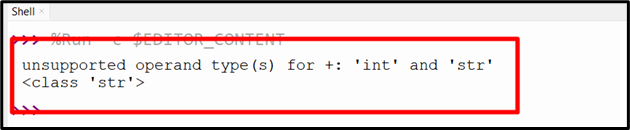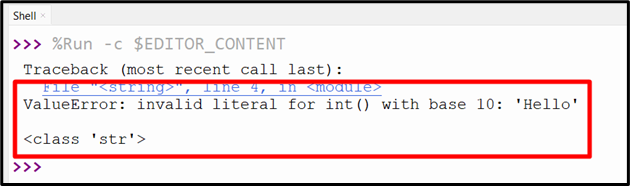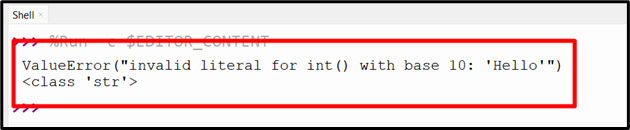| by Arround The Web | No comments

## How Do I Convert an Exception to a String in Python

An “exception” is a circumstance that disrupts the normal flow of instructions throughout the execution of a program. Python stops executing a program/code when an exception is thrown. If you’re a Python developer, you’ve probably run into errors or exceptions in your code. Python provides various methods to handle these exceptions with the “try-except” block. However, sometimes you may want to convert an exception to a string to get more information about the error.

In this Python article, we’ll explore the approaches to converting an exception to a Python string.

## How to Convert an Exception to a Python String?

To convert an exception to a string, apply the following approaches:

## Method 1: Convert an Exceptions to a String in Python Using the “str()” Function

The simplest way to convert an exception to a string is via the built-in “str()” function. This function accepts an object as an argument and retrieves a string representation of the object. It returns an error message when the exception object is passed as an argument.

Syntax

str(object)

Here, “object” is any Python object that can be converted to a string representation. The “str()” function returns a string object.

Example

The following example illustrates the discussed concept:

try:
print(1 + '3')
except Exception as e:
error_message = str(e)
print(error_message)
print(type(error_message))

In the above code, the “TypeError” exception is caught by the “except” block. The “except” block assigns the exception object to the variable “e” and it is then passed to the “str()” function as an argument that converts it to a string.

OutputIn the above output, the exception has been converted into a string appropriately.

## Method 2: Convert an Exceptions to a String in Python Using “traceback.format_exc()” Function

The “traceback.format_exc()” function of the “traceback” module can also be used to convert an exception to a string. This function returns a formatted traceback for the last exception that occurred. In the traceback, you will find/get the error message, the location where the error occurred, and the function call stack.

Syntax

traceback.format_exc(limit=None, chain=True)

In the above syntax:

• The “limit (optional)” parameter indicates the number of stack levels to show for each exception in the traceback. The default value “None” means that all levels are shown.
• The “chain” parameter is optional and its default value is “true”. When set to “true”, the function prints the full traceback of the exception and all exceptions in the chain. Setting it to “false” prints only the traceback of the most recent exception.

Example

As an example, let’s look at the following code snippet:

import traceback
try:
value = 'Hello'
print(int(value))
except Exception as e:
error_message = traceback.format_exc()
print(error_message)
print(type(error_message))

According to the above code, the “try-except” block is used to handle the exception and print the error message using the “traceback” module. The “traceback.format_exc()” function retrieves a string that contains the stack trace of the exception.

OutputThis outcome indicates that the exception has been successfully converted into a string.

## Method 3: Convert an Exceptions to a String in Python Using the “repr()” Function

In Python, the “repr()” function is used to return a printable representation of an object in Python. This function can also be applied to convert an exception into a string.

Syntax

repr(object)

In this syntax, “object” is any Python object whose printable representation needs to be returned.

Example

Consider the following example code:

try:
value = 'Hello'
print(int(value))
except Exception as e:
error_message = repr(e)
print(error_message)
print(type(error_message))

In the above code block, the “repr()” function is used in the “except” block to catch the faced exception and convert it into a string.

OutputAccording to the above output, the faced exception has been converted into a string.

## Conclusion

To convert an exception to a string in Python, apply the “str()” function, “traceback.format_exc()” function, or the “repr()” function. All of these functions are used combined with the “try-except” blocks to catch and convert the faced exception into a string. This guide presented various methods to convert an exception to a string in Python using numerous examples.

Source: linuxhint.com